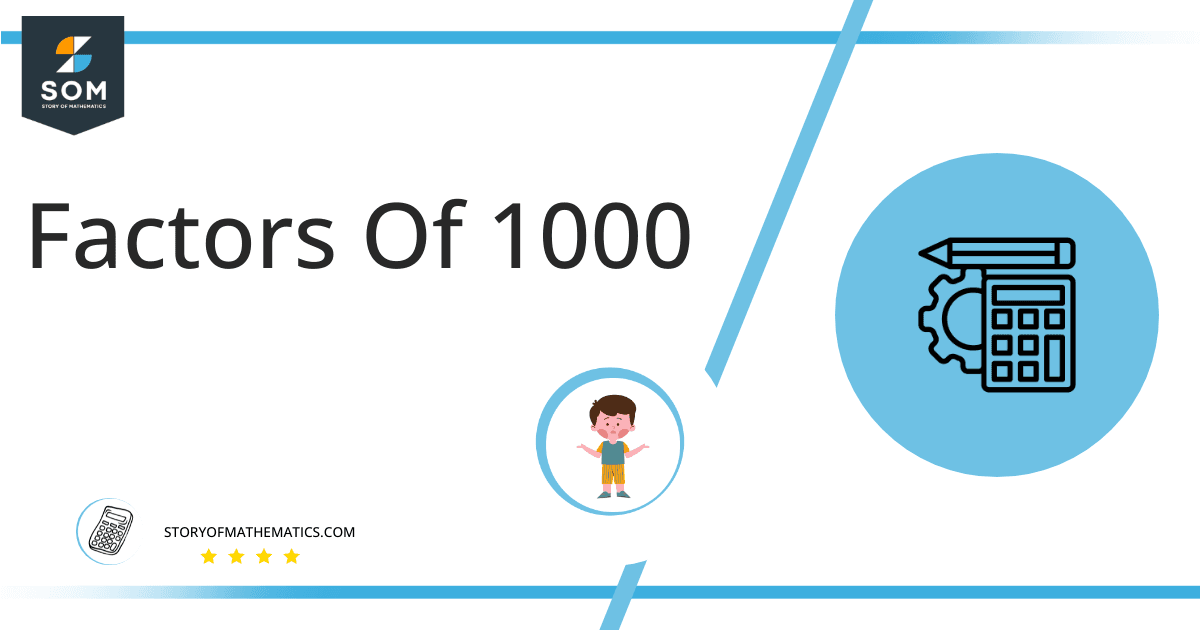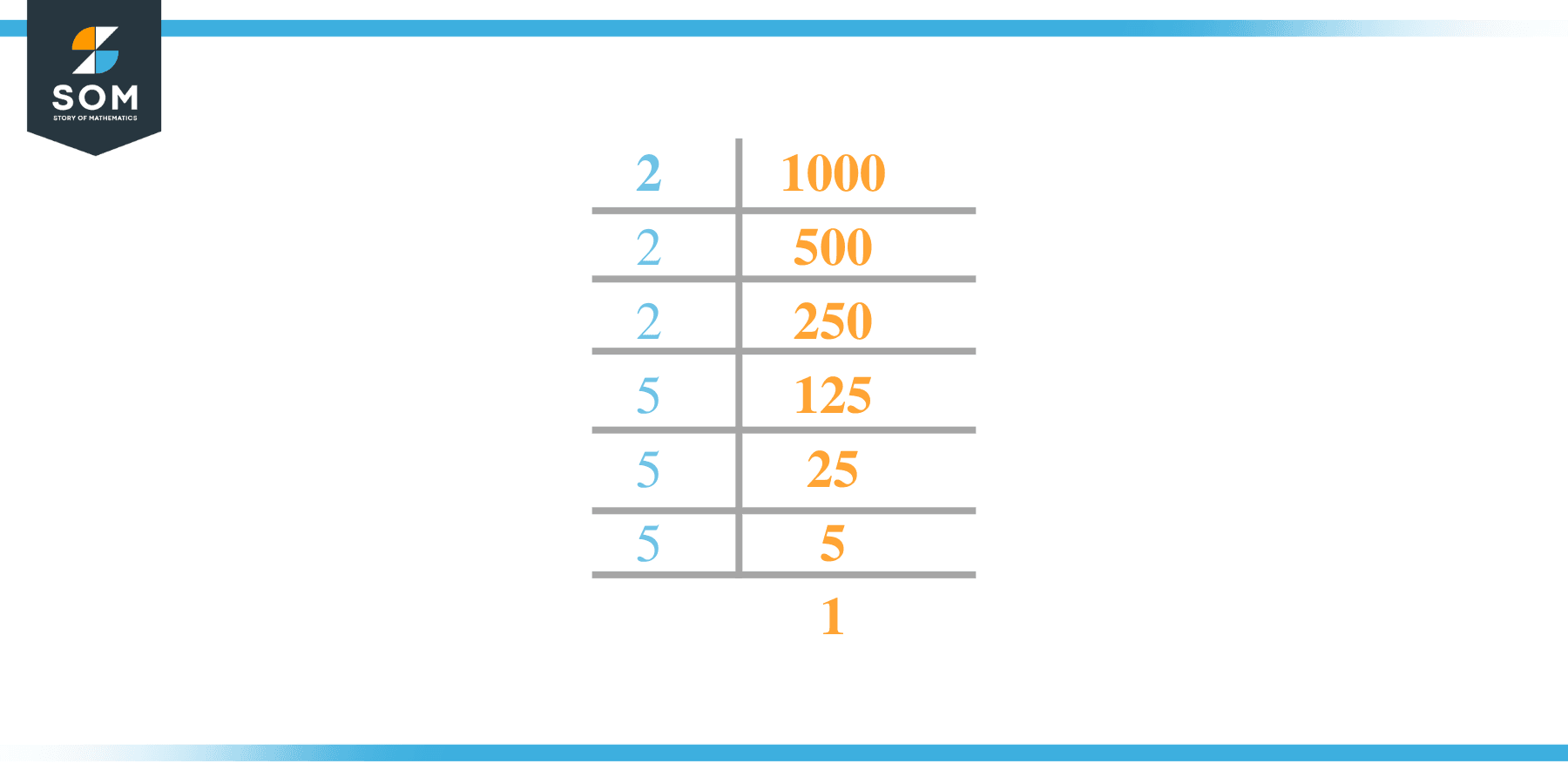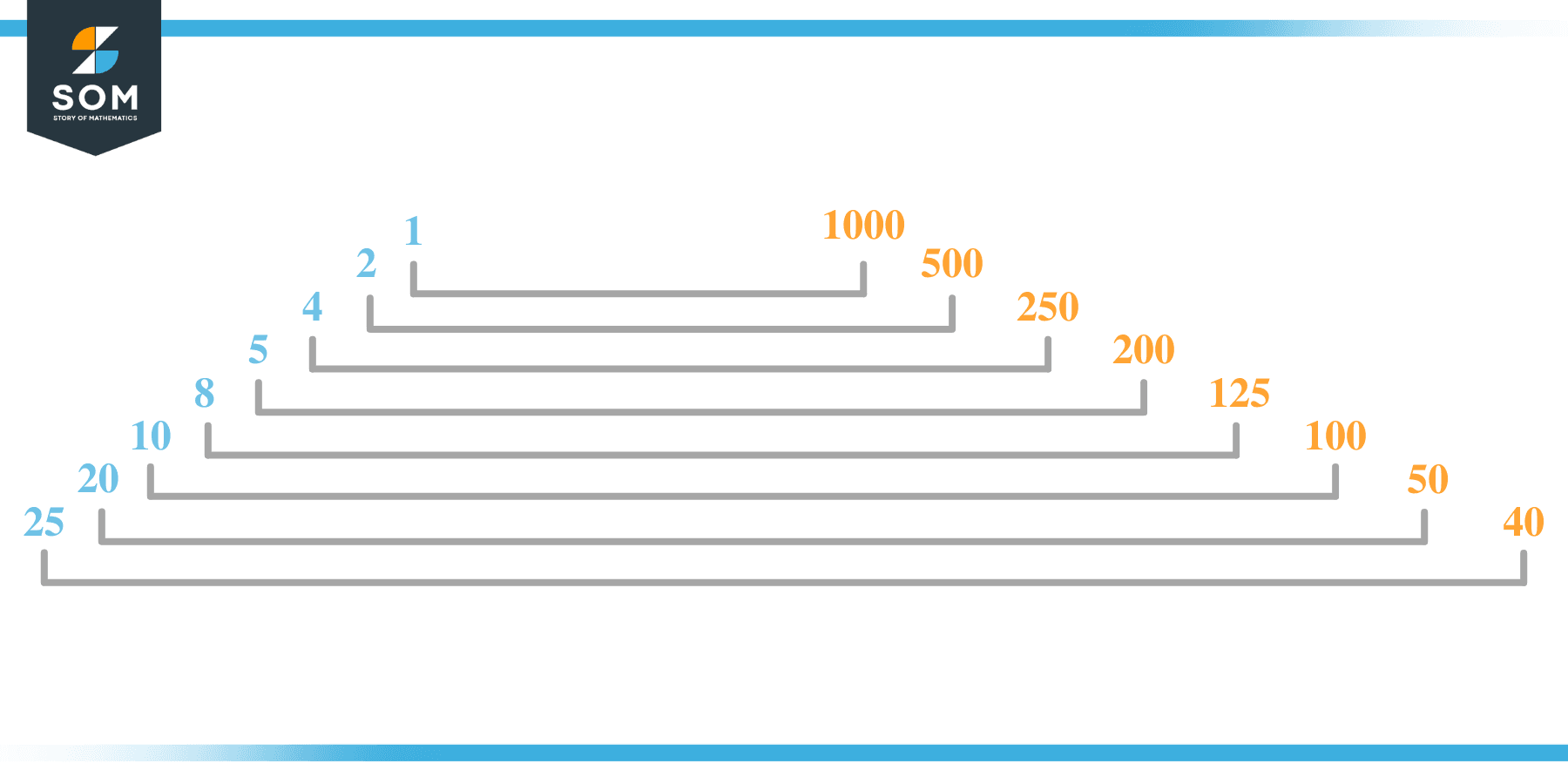# Factors of 1000: Prime Factorization, Methods, and Examples

Factors are the numbers that evenly divide a given number. The given number 1000 is a composite number and has overall 16 factorsThis article not only determines the factors and prime factors of 1000 but also provides the method and examples to explain them.

### Factors of 1000

Here are the factors of number 1000.

Factors of 1000: 1, 2, 4, 5, 8, 10, 20, 25, 40, 50, 100, 125, 200, 250, 500, and 1000

### Negative Factors of 1000

The negative factors of 1000 are similar to its positive aspects, just with a negative sign.

Negative Factors of 1000: -1, -2, -4, -5, -8, -10, -20, -25, -40, -50, -100, -125, -200, -250, -500, and -1000

### Prime Factorization of 1000

The prime factorization of 1000 is the way of expressing its prime factors in the product form.

Prime Factorization: 2 × 2 × 2 × 5 × 5 × 5

In this article, we will learn about the factors of 1000 and how to find them using various techniques such as upside-down division, prime factorization, and factor tree.

## What Are the Factors of 1000?

The factors of 1000 are 1, 2, 4, 5, 8, 10, 20, 25, 40, 50, 100, 125, 200, 250, 500, and 1000. These numbers are the factors as they do not leave any remainder when divided by 1000.

The factors of 1000 are classified as prime numbers and composite numbers. The prime factors of the number 1000 can be determined using the prime factorization technique.

## How To Find the Factors of 1000?

You can find the factors of 1000 by using the rules of divisibility. The divisibility rule states that any number, when divided by any other natural number, is said to be divisible by the number if the quotient is the whole number and the resulting remainder is zero.

To find the factors of 1000, create a list containing the numbers that are exactly divisible by 1000 with zero remainders. One important thing to note is that 1 and 1000 are the 1000’s factors as every natural number has 1 and the number itself as its factor.

1 is also called the universal factor of every number. The factors of 1000 are determined as follows:

$\dfrac{1000}{1} = 1000$

$\dfrac{1000}{2} = 500$

$\dfrac{1000}{4} = 250$

$\dfrac{1000}{5} = 200$

$\dfrac{1000}{8} = 125$

$\dfrac{1000}{10} = 100$

$\dfrac{1000}{20} = 50$

$\dfrac{1000}{25} = 40$

$\dfrac{1000}{1000} = 1$

Therefore, 1, 2, 4, 5, 8, 10, 20, 25, 40, 50, 100, 125, 200, 250, 500, and 1000 are the factors of 1000.

### Total Number of Factors of 1000

For 1000, there are 16 positive factors and 16 negative ones. So in total, there are 32 factors of 1000.

To find the total number of factors of the given number, follow the procedure mentioned below:

1. Find the factorization/prime factorization of the given number.
2. Demonstrate the prime factorization of the number in the form of exponent form.
3. Add 1 to each of the exponents of the prime factor.
4. Now, multiply the resulting exponents together. This obtained product is equivalent to the total number of factors of the given number.

By following this procedure, the total number of factors of 1000 is given as:

Factorization of 1000 is 1 × 2 × 2 × 2 × 5 × 5 × 5.

The exponent of 1, 2, and 5 is 1, 3, and 3 respectively.

Adding 1 to each and multiplying them together results in 32.

Therefore, the total number of factors of 1000 is 32. 16 are positive, and 16 factors are negative.

### Important Notes

Here are some essential points that must be considered while finding the factors of any given number:

• The factor of any given number must be a whole number.
• The factors of the number cannot be in the form of decimals or fractions.
• Factors can be positive as well as negative.
• Negative factors are the additive inverse of the positive factors of a given number.
• The factor of a number cannot be greater than that number.
• Every even number has 2 as its prime factor, the smallest prime factor.

## Factors of 1000 by Prime FactorizationThe number 1000 is a composite number. Prime factorization is a valuable technique for finding the number’s prime factors and expressing the number as the product of its prime factors.

Before finding the factors of 1000 using prime factorization, let us find out what prime factors are. Prime factors are the factors of any given number that are only divisible by 1 and themselves.

To start the prime factorization of 1000, start dividing by its most minor prime factor. First, determine that the given number is either even or odd. If it is an even number, then 2 will be the smallest prime factor.

Continue splitting the quotient obtained until 1 is received as the quotient. The prime factorization of 1000 can be expressed as:

1000 = 2 × 2 × 2 × 5 × 5 × 5

## Factors of 1000 in PairsThe factor pairs are the duplet of numbers that, when multiplied together, result in the factorized number. Factor pairs can be more than one depending on the total number of factors given.

For 1000, the factor pairs can be found as:

1 x 1000 = 1000

2 x 500 = 1000

4 x 250 = 1000

5 x 200 = 1000

8 x 125 = 1000

10 x 100 = 1000

20 x 50 = 1000

25 x 40 = 1000

The possible factor pairs of 1000 are given as (1, 1000), (2, 500), (4, 250), (5, 200), (8, 125), (10, 100), (20, 50), and (25, 40).

All these numbers in pairs, when multiplied, give 1000 as the product.

The negative factor pairs of 1000 are given as:

-1 x -1000 = 1000

-2 x -500 = 1000

-4 x -250 = 1000

-5 x -200 = 1000

-8 x -125 = 1000

-10 x -100 = 1000

-20 x -50 = 1000

-25 x -40 = 1000

It is important to note that in negative factor pairs, the minus sign has been multiplied by the minus sign, due to which the resulting product is the original positive number. Therefore, -1, -2, -4, -5, -8, -10, -20, -25, -40, -50, -100, -125, -200, -250, -500, and -1000 are called negative factors of 1000.

The list of all the factors of 1000, including positive as well as negative numbers, is given below.

Factor list of 1000: 1, -1, 2, -2, 4, -4, 5, -5, 8, -8, 10, -10, 20, -20, 25, -25, 40, -40, 50, -50, 100, -100, 125, -125, 200, -200, 250, -250, 500, -500, 1000, and -1000

## Factors of 1000 Solved Examples

To better understand the concept of factors, let’s solve some examples.

### Example 1

How many factors of 1000 are there?

### Solution

The total number of Factors of 1000 is 32.

Factors of 1000 are 1, 2, 4, 5, 8, 10, 20, 25, 40, 50, 100, 125, 200, 250, 500, and 1000.

### Example 2

Find the factors of 1000 using prime factorization.

### Solution

The prime factorization of 1000 is given as:

1000 $\div$ 2 = 500

500 $\div$ 2 = 250

250 $\div$ 2 = 125

125 $\div$ 5 = 25

25 $\div$ 5 = 5

5 $\div$ 5 = 1

So the prime factorization of 1000 can be written as:

2 × 2 × 2 × 5 × 5 × 5 = 1000